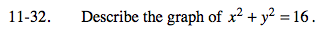Home > PC > Chapter 11 > Lesson 11.1.2 > Problem11-32

11-32.

Describe the graph of x2 + y2 = 16. Homework Help ✎The equation is in the form x2 + y2 = r2. What is the shape of the graph?

This is the graph of a circle with center (_, _) and radius _.